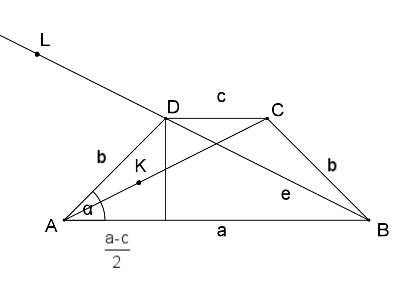Mathematical and Physical Journal
for High Schools
Issued by the MATFUND Foundation
 Already signed up? New to KöMaL?

#Problem C. 974. (January 2009)

C. 974. ABCD is a cyclic trapezium. Diagonal BD is extended beyond vertex D by the length of the leg and the point L is obtained. K is the point on diagonal AC whose distance from C is equal to the leg. Prove that the areas of the rectangles formed by the line segments AB and CD and the line segments AK and BL are equal.

(5 pont)

Deadline expired on February 16, 2009.

Sorry, the solution is available only in Hungarian. Google translation

Megoldás. Igazolandó: AB.CD=AK.BL, a szokásos jelölésekkel: ac=(e-b)(e+b). Ezt így is írhatjuk: e2=b2+ac. Az ADC háromszögben a koszinusz-tétel: e2=b2+c2-2bccos (180o-). Elegendő lenne bizonyítani, hogy c2-2bccos (180o-)=ac, azaz: c-2bcos (180o-)=a, vagyis, illetve. Ez pedig igaz.### Statistics:

 179 students sent a solution. 5 points: 164 students. 4 points: 4 students. 3 points: 4 students. 2 points: 2 students. 1 point: 1 student. 0 point: 1 student. Unfair, not evaluated: 3 solutionss.

Problems in Mathematics of KöMaL, January 2009